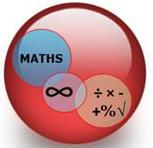## Maths Across the Curriculum 'M.A.C.'M.A.C. stands for 'Maths Across the Curriculum' and with the appointment of our Leading Maths Practitioner & Associate Senior Leader, Mrs Kainth, back in Jan 2016, the school has enjoyed a new focus and drive for improvement on mathematical skills over the past years.

Along with Literacy & Communication, M.A.C. skills are the key stone for effective learning and progression in school and important life skills. This is why our pupils experience a weekly focus on literacy for half a term and then a weekly focus on mathematical skills.  This is introduced in tutor time and reinforced during the week in our lessons.

The M.A.C. logo is publicised across the school and used in teaching powerpoints and resources across the curriculum so that teachers can highlight mathematical teaching points during the course of their lessons and schemes of work.  Each department has undergone an audit to reflect on their contribution to the M.A.C. curriculum and this information has been used to create posters & puzzles for classrooms across the school.

In addition, we are delighted to share this parent/carer handbook which has been written especially with parents in mind.  Sometimes

mathematical techniques change over time and we thought it would be useful to share the techniques being used at Toynbee so that there is a consistency of approach and awareness of the way pupils are required to work.  We hope you find this useful.

We also offer  mentoring scheme with year 7 and 9 pupils. This scheme aims to help build Year 7 pupils’ confidence

in Maths and to make further progress in the subject with support from Year 9 Maths Leaders with whom they have been paired.  As with Reading Mentors, we have found that peer learning and support is a one of the most effective ways of making gains in understanding.

Mathematical terms - Keywords

• Calculate - A numerical answer is expected which requires the use of a calculator or formal calculation methods

• Deduce - Use logic; one step follows on from another and reasoning is given.

• Describe fully - Use mathematical vocabulary in the description.  Ensure that the answer is unambiguous

• Do an accurate drawing - Use compasses to draw lengths (rather than just a ruler), protractors for measuring angles and a sharp pencil to avoid parallax errors.

• Estimate - An estimate is not a guess.  Use rounded numbers to work out an approximate number.

• Expand - Multiply to convert the brackets into the individual algebraic or numerical terms.

• Expand & simplify - Multiply to convert the brackets and then collect terms together to form the simplest version possible.

• Explain/Comment/Give a reason for your answer - Use words and/or mathematical symbols to justify an answer or describe the limits of the process you have used to reach your answer.

• Express, in terms of - Use the given information to write an expression which only uses the letter(s) given

• Factors - Factors are numbers which can be multiplied together to get another number

• Give the answer to a sensible degree of accuracy for scientists - Answers should be no more accurate than the values given in the

• question.  If the question quotes values to 2 sf. Then give an answer to either 2sf. Or 1sf.

• Hence - Use the previous answer to work out the next part.

• Make (x) the subject - Rearrange a formula so that it is written x =

• Measure - Use a ruler or a protractor to measure a length or an angle.

• Product - The answer when two or more numbers are multiplied together

• Prove - An algebraic or geometric proof is required where each step is justified

• Show that - Use reasoning which utilises words, numbers or algebra to find an answer

• Simplify - Collect algebraic terms together or cancel down a fraction to find the simplest version of an answer

• Solve - Find the value(s) of (x) that makes the equation true.

• Use the graph - Do not calculate the answer, read the answer from the graph.  Draw marker lines on the graph to show the answer has

• been found.

• Work Out - A calculation is involved in finding the answer